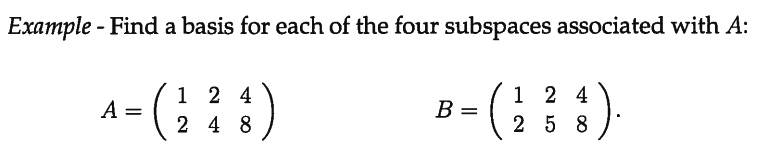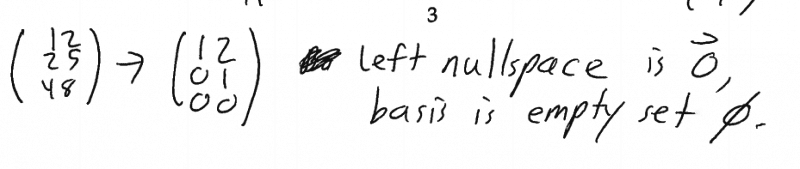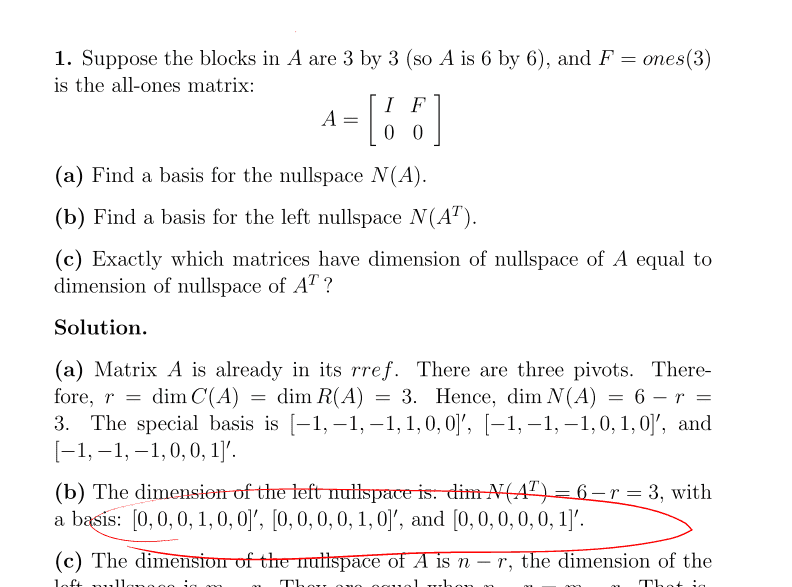# How they found the left nullspace in each of these examples

• LongApple

## Homework Statement

Part b)

http://www.math.utah.edu/~zwick/Classes/Fall2012_2270/Lectures/Lecture19_with_Examples.pdfFor BLeft nullspace is solution to A ^ T times Y =0
So we have a free variable for the third row so don't we have infinitely many solutions as x3 could be anything?In this problem's part b) I don't think that they took the transpose of the matrix A.

http://staff.imsa.edu/~fogel/LinAlg/PDF/29 Fundamental Subspaces.pdf
Go the bottom of page 1 under 4) and you'll see "
Perform Gaussian (or better, Gauss-Jordan) elimination on [A | I] to produce [U | B] (or
[R | C]). Claim: the last m – r rows of B (which equal those of C because once we get zeroes
there’s no more work to do in those rows) form ..."

So why isn't the last three rows of 0's in A just the left nullspace?

I am trying to figure out why

## The Attempt at a Solution

#### Attachments

LongApple said:
Left nullspace is solution to A ^ T times Y =0
So we have a free variable for the third row so don't we have infinitely many solutions as x3 could be anything?
B is a 2x3 matrix, so it maps vectors from ##\mathbb{R}^3## to ##\mathbb{R}^2##. In which of these two vector spaces does the left nullspace reside?

In this problem's part b) I don't think that they took the transpose of the matrix A.

http://staff.imsa.edu/~fogel/LinAlg/PDF/29 Fundamental Subspaces.pdf
Go the bottom of page 1 under 4) and you'll see "
Perform Gaussian (or better, Gauss-Jordan) elimination on [A | I] to produce [U | B] (or
[R | C]). Claim: the last m – r rows of B (which equal those of C because once we get zeroes
there’s no more work to do in those rows) form ..."

So why isn't the last three rows of 0's in A just the left nullspace?
You start with the (untransposed) A and form the augmented matrix [A | I] and perform row operations to produce [U | B]. The basis vectors are the last m-r rows of B. So why are you thinking the rows of A have anything to do with the basis of the left nullspace? It should be clear the last three rows of A can't form basis because they're all 0s.

vela said:
B is a 2x3 matrix, so it maps vectors from ##\mathbb{R}^3## to ##\mathbb{R}^2##. In which of these two vector spaces does the left nullspace reside?

You start with the (untransposed) A and form the augmented matrix [A | I] and perform row operations to produce [U | B]. The basis vectors are the last m-r rows of B. So why are you thinking the rows of A have anything to do with the basis of the left nullspace? It should be clear the last three rows of A can't form basis because they're all 0s.

I don't think so.

But then what does this mean?